# SAT I Math — What’s New?

By Sara VanceIf you have taken a look at the math sections on the new, re-designed SAT I math tests that the College Board has released, you will see that there are some new concepts and some new math words and phrases that are showing up frequently.

Here are some that you should review and note how the College Board incorporates them into their math questions.

## Dividing Polynomials

Division of one polynomial by another is done using long division. The terms to be divided must be arranged in decreasing order, and a place marker must be made for every omission in degree.

Here are two examples: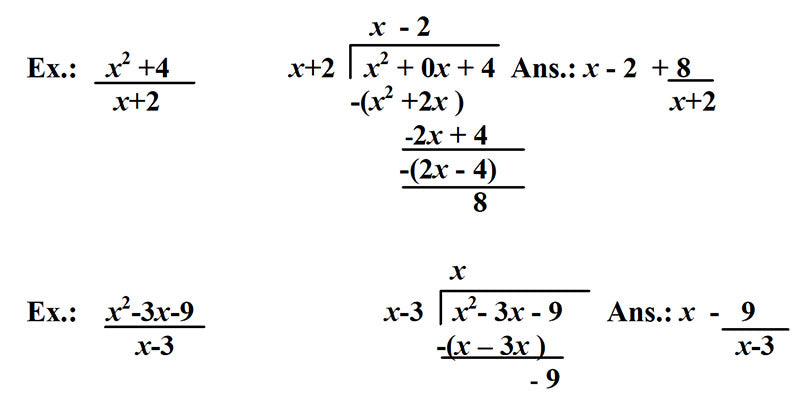## Synthetic Division

Another faster way to answer this type of question is to do Synthetic Division. Study this example to review the process for synthetic division: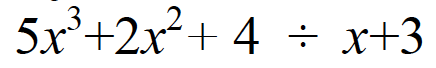Ans.: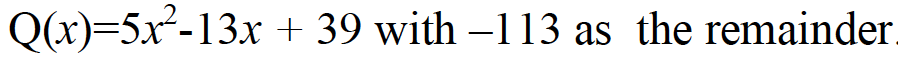Synthetic division is used when one of the coefficients of the terms in the polynomial is asked for:What is k?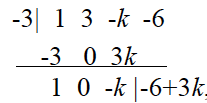Since to be a factor the division can have no remainder. Therefore, 3k-6 must equal 0. k = 2 .

## Factors of Polynomials

In the division problems above, there are remainders so you know the divisors x+2 and x-3 are not factors of the polynomialsThe term factor is another term that appears frequently on the new test. Remember: to be a factor, there can be no remainder. Therefore, if f(-7)=0, then (x+7) is a factor of the function. But if f(-7)=5, then (x+7) cannot be a factor.

Ex.: If in the quadratic function f, f(6) = 0 and f(-2) = 0, what is the minimum value of function f ?

Since 6 and –2 are the zeros of the function, (x-6) and (x+2) are factors of the quadratic function. The minimum value is the y-value when x = -b/2a, or 2. f(2) = -16.

Ex.: Is (x + 1) a factor of f(x) = x11 –4x9 –3x - 8 ?

By plugging in –1 to the function, f(-1) = 0, so (x+1) is a factor of function f.

## Expected Total Outcome

When a problem asks for the expected total number or expected outcome, in most cases direct proportions is the math operation required.

Ex.: A city of with a population of 42,500 is considering building a new community center. It randomly contacted 350 citizens from all areas of the city to ask whether they would be in favor of the new center. The results are shown in the table below. What is the approximate expected number of citizens not in favor of the new center?## Co-Functions

There are 6 trig cofunctions:Any trig function is equal to the cofunction of the complement of the angle: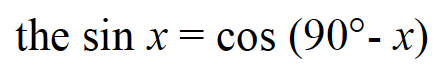## Quadratics: the Sum and Product of the Roots

In a parabola,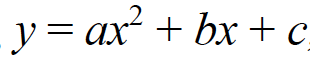the sum of the roots = –b/a , and the product of the roots = c/a .

Ex.: What is the sum and product of all the values of x that satisfy the equation,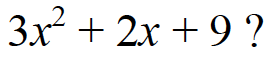Ans.: sum = -2/3 product = 9/3 or 3

More than likely, the roots of the parabola that is given will not be found by factoring, so using these formulas will be faster than either graphing or using the quadratic formula to find the answer.

# WANT TO RAISE YOUR SCORE?

## MASTER THE SAT I REDESIGNED MATH TEST

to know all of the SAT 1 Math formulas and processes is by mastering the AdVANCE SAT I Math Attack flashcards in combination with the practice tests available in the text published by The College Board. The flashcards have helped hundreds of students dramatically raise their scores!

## Factoring the Sum and Difference of 2 Cubes

On the redesigned SAT I test, the student must be able to factor the sum and difference of cubes.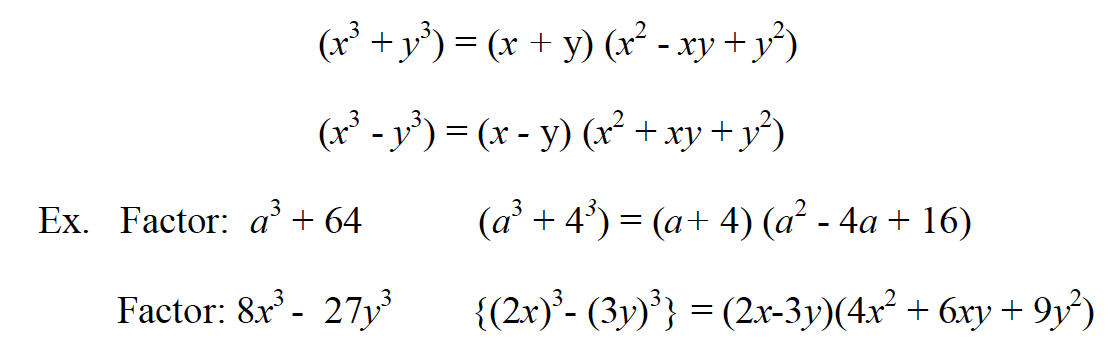## Factoring by Grouping

On the redesigned SAT I test, the student must be able to factor polynomial expressions using the grouping method. Below are some examples.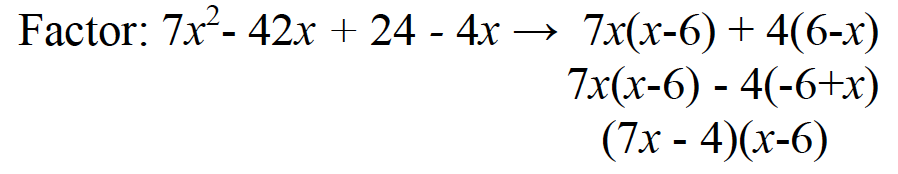Note: when factors are opposites of each other, a -1 must be factored out to make them the same.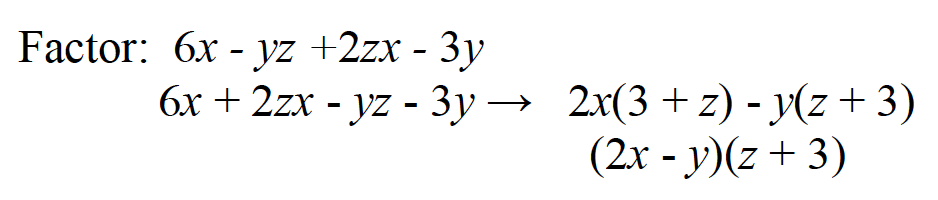Note: the terms may have to be rearranged before factoring.Note: in addition to grouping as two binomials, grouping can be as a trinomial and a monomial if the trinomial is a trinomial square. Doing so creates the difference of 2 squares.

## When the Product of 2 Binomials Equals a Trinomial

In quadratic problems involving trinomials, if the square of a binomial is equal to a degree 2 trinomial, then once the binomial square is expanded, each part of the binomial is equal to the term of corresponding degree in the trinomial.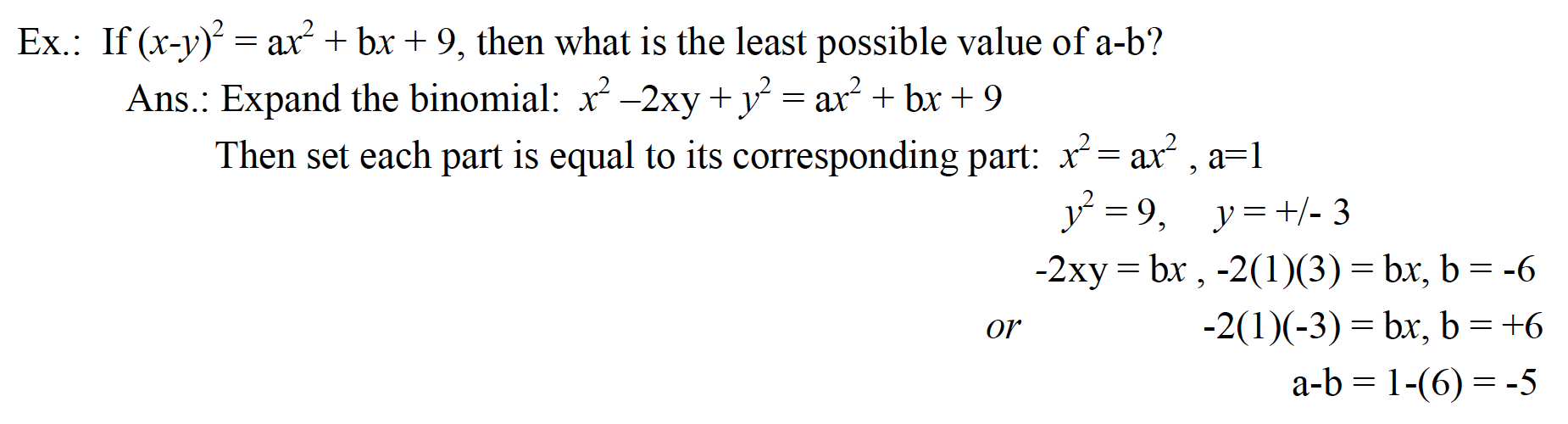A circle contains 360°. In the unit circle, since the radius = 1, the circumference (360°) is equal to 2π. Therefore, to convert an angle from the degree form to radian form, set up a direct proportion:## Growth / Decay Functions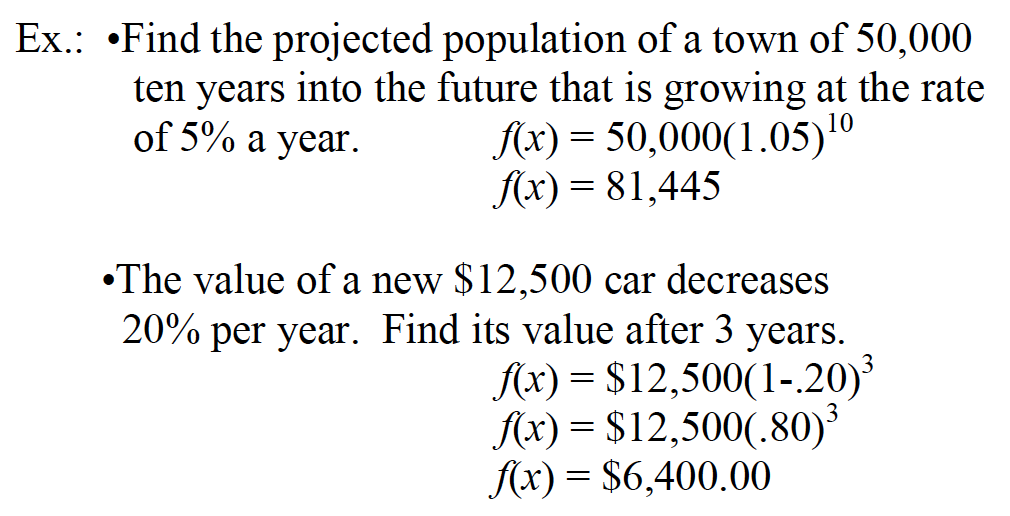## Systems of Linear Equations

The re-designed SAT I math contains more linear systems questions that focus on slope and on systems that have infinite number of solutions or no solution. You should know the following:

• The slopes of linear systems with no solution are equal; the lines are parallel.
• The slopes of linear systems that have exactly one solution are not equal; the lines intersect.
• The slopes of coinciding lines are equal, and their y-intercepts are also equal.
• The slopes of perpendicular lines are negative reciprocals; the lines intersect.

Ex.: If the following linear system has infinite solutions, with a and b as constants, what is the value of a + b?

2x + ay = 19
bx + y = 3.8

Since the lines must coincide to have infinite solutions, the coefficients of the x and y terms must be equal, as well as the y-intercepts. If the first equation is multiplied by .2, the y-intercepts will be the same:

4x + .2ay = 3.8. So then: .4x + .2ay = 3.8
bx + y = 3.8

Since the coefficients must also be equal, b = .4 and .2a =1, a=5, and a + b = 5.4

## A Constant

Another term showing up on the new test is a constant. Constants are part of algebraic expressions that do not change; they are fixed terms. For example, in the equation, 2x + 5 = 8, 5 and 8 are constants. If a term is not a constant, then it is a variable (2x is a variable term).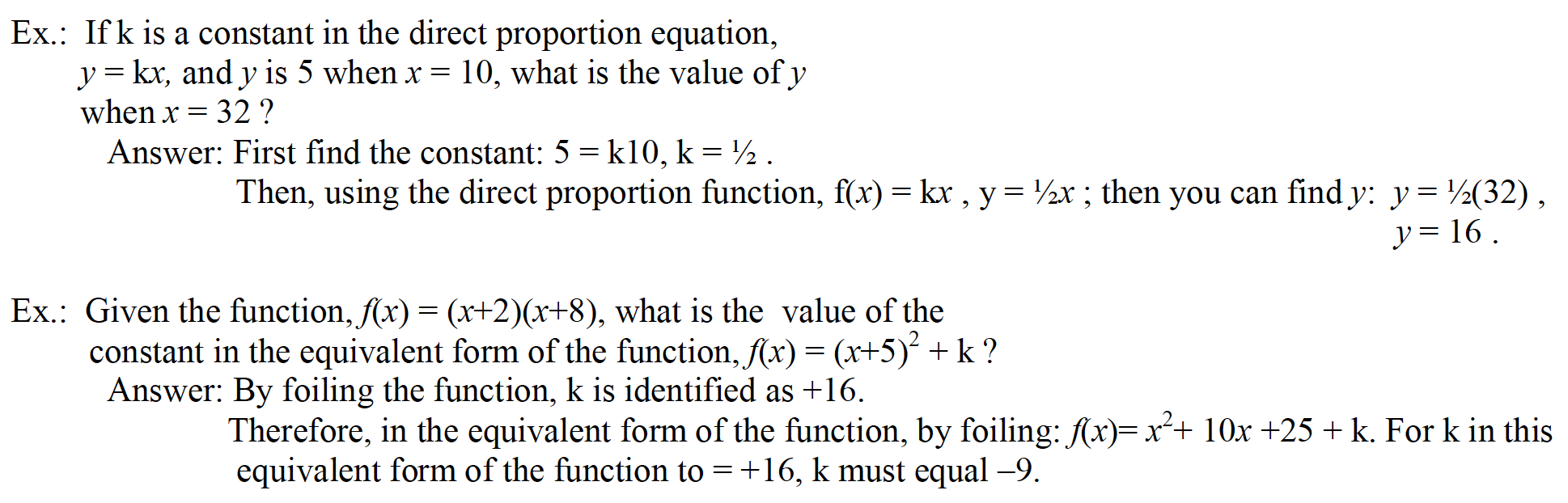## Complex Numbers

A complex number is a number in the form a + bi, where a and b are real numbers and i is the imaginary part.

Two complex numbers are equal only if they have equal real parts and equal imaginary parts:

If x + yi = 4-i , then x = 4 and y = -1 .

To add, you add the real parts and then the imaginary parts: (4 + 3i) + (2 + 4i) = 6 + 7i

You must know how to simplify the quotient of a complex number. The conjugate of the denominator is used to rationalize the denominator: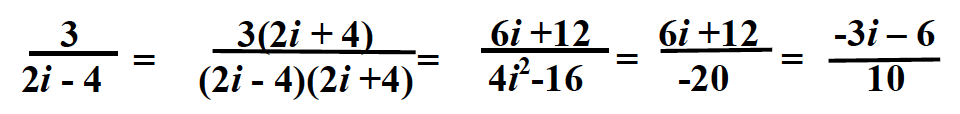## Graphs of Polynomials

Odd-powered polynomial f(x)’s with a positive leading coefficient, such aswill always be down on the left and up on the right side of the graph.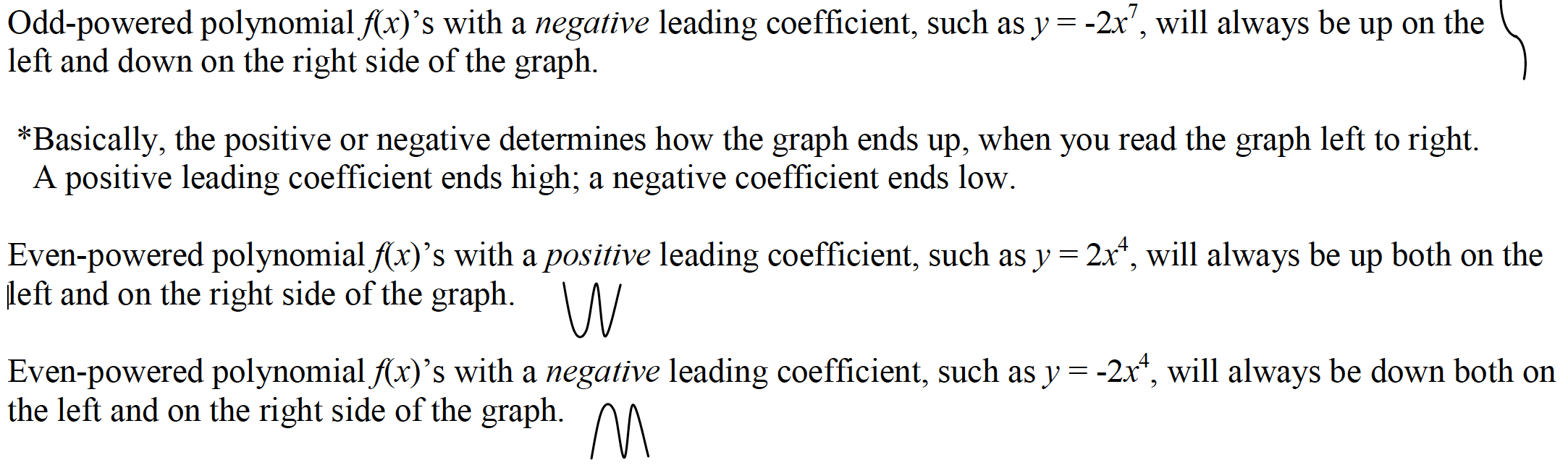## Standard of Deviation

The standard of deviation is the measure of how far from the mean (average) the data deviates. To date, the College Board is not requiring the student to actually find the standard of deviation, the it is important to have a general understanding of the concept. To find the standard of deviation:

1. First, find the mean (average) of the data set.
2. Then find the sum of the squares of the deviations from this mean.
3. Next, divide this sum by the number of terms in the set.
4. Finally, take the square root of this quotient.

Basically, standard of deviation is a measure of how spread out the numbers are.

Example: What is the standard of deviation of the following distribution: 8, 10, 13, 14, 15 ?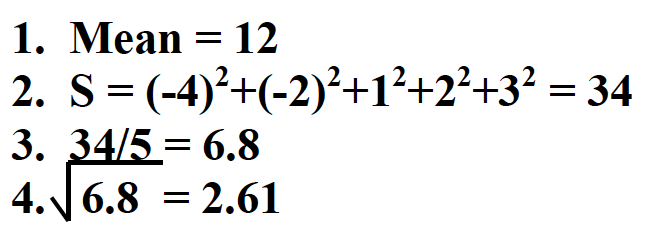## Parabolas: Discriminants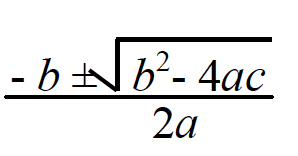The discriminant is not used to determine the actual roots, but rather the nature of the roots.Ok, now the rest is up to you. GOOD LUCK! If we can answer any questions, feel free to contact us.

# WANT TO RAISE YOUR SCORE?

## MASTER THE SAT I REDESIGNED MATH TEST

to know all of the SAT 1 Math formulas and processes is by mastering the AdVANCE SAT I Math Attack flashcards in combination with the practice tests available in test prep materials published by The College Board. The flashcards have helped hundreds of students dramatically raise their scores!